Examples

Chapter 9 Class 11 Sequences and Series
Serial order wise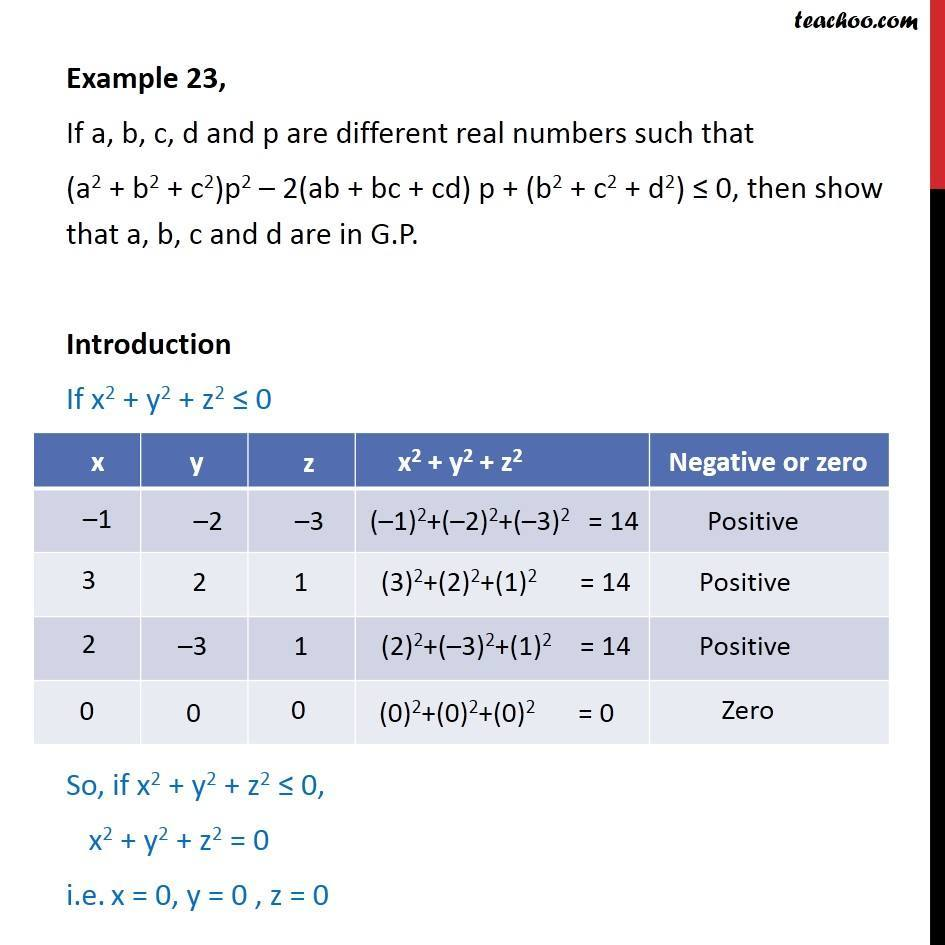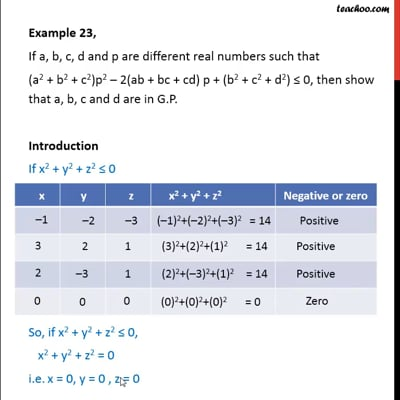This video is only available for Teachoo black users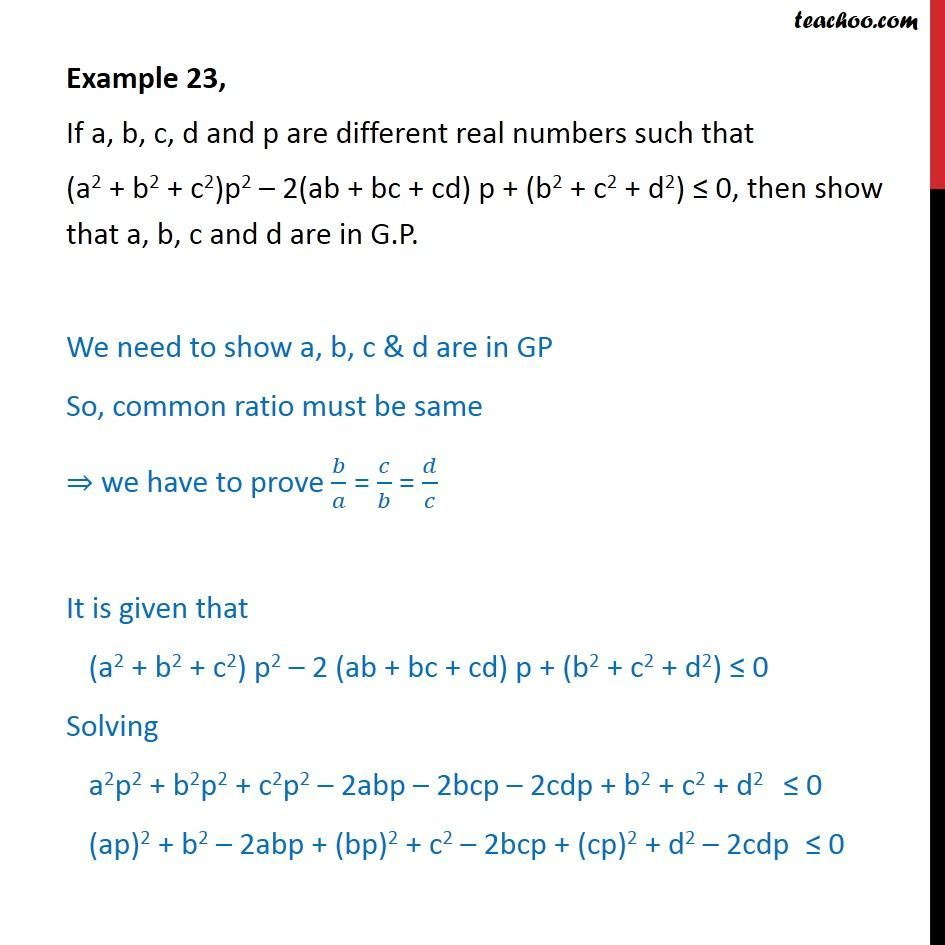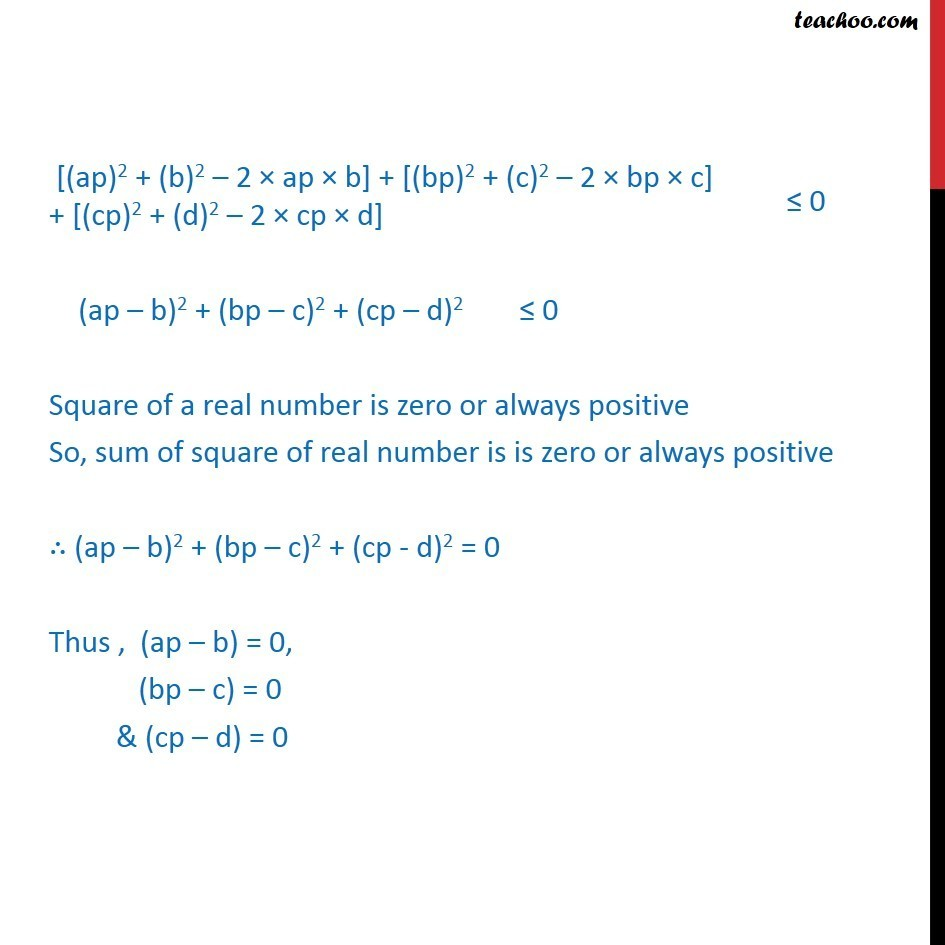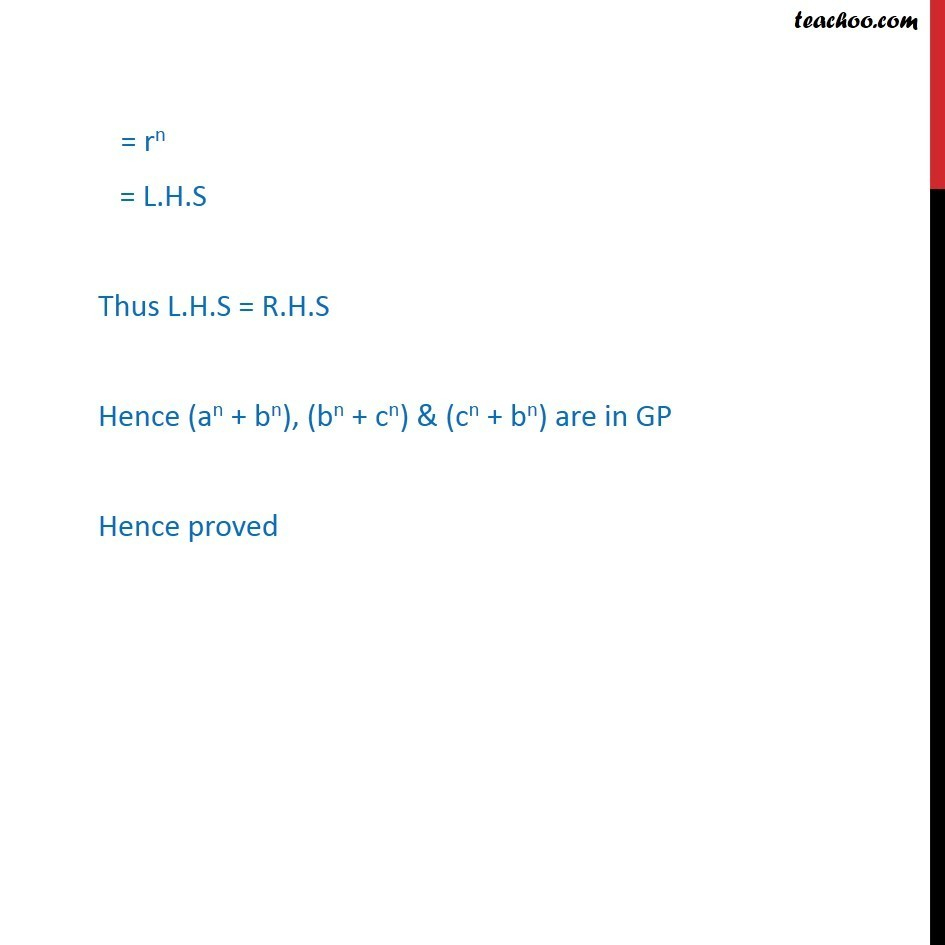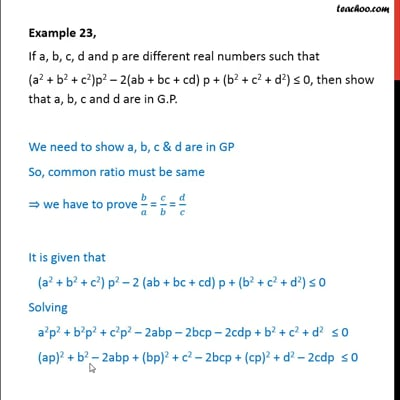This video is only available for Teachoo black users

Solve all your doubts with Teachoo Black (new monthly pack available now!)

### Transcript

Example 23, If a, b, c, d and p are different real numbers such that (a2 + b2 + c2)p2 2(ab + bc + cd) p + (b2 + c2 + d2) 0, then show that a, b, c and d are in G.P. Introduction If x2 + y2 + z2 0 So, if x2 + y2 + z2 0, x2 + y2 + z2 = 0 i.e. x = 0, y = 0 , z = 0 Example 23, If a, b, c, d and p are different real numbers such that (a2 + b2 + c2)p2 2(ab + bc + cd) p + (b2 + c2 + d2) 0, then show that a, b, c and d are in G.P. We need to show a, b, c & d are in GP So, common ratio must be same we have to prove / = / = / It is given that (a2 + b2 + c2) p2 2 (ab + bc + cd) p + (b2 + c2 + d2) 0 Solving a2p2 + b2p2 + c2p2 2abp 2bcp 2cdp + b2 + c2 + d2 0 (ap)2 + b2 2abp + (bp)2 + c2 2bcp + (cp)2 + d2 2cdp 0 Example 23, If a, b, c, d and p are different real numbers such that (a2 + b2 + c2)p2 2(ab + bc + cd) p + (b2 + c2 + d2) 0, then show that a, b, c and d are in G.P. We need to show a, b, c & d are in GP So, common ratio must be same we have to prove / = / = / It is given that (a2 + b2 + c2) p2 2 (ab + bc + cd) p + (b2 + c2 + d2) 0 Solving a2p2 + b2p2 + c2p2 2abp 2bcp 2cdp + b2 + c2 + d2 0 (ap)2 + b2 2abp + (bp)2 + c2 2bcp + (cp)2 + d2 2cdp 0 Solving (ap b) = 0 ap = b / = p Solving (bp c) = 0 bp = c / = p Solving (cp d) = 0 cp = d / = p This implies that (b )/a = (c )/b = (d )/c = p Hence a, b, c and d are in G.P.